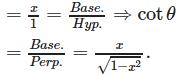# Test: Inverse Trigonometry -1

## 25 Questions MCQ Test Mathematics (Maths) Class 12 | Test: Inverse Trigonometry -1

Description
This mock test of Test: Inverse Trigonometry -1 for JEE helps you for every JEE entrance exam. This contains 25 Multiple Choice Questions for JEE Test: Inverse Trigonometry -1 (mcq) to study with solutions a complete question bank. The solved questions answers in this Test: Inverse Trigonometry -1 quiz give you a good mix of easy questions and tough questions. JEE students definitely take this Test: Inverse Trigonometry -1 exercise for a better result in the exam. You can find other Test: Inverse Trigonometry -1 extra questions, long questions & short questions for JEE on EduRev as well by searching above.
QUESTION: 1

### If ƒ(x) = √(2tan(x)),then f-1(√(2)) =

Solution:

Explanation : ƒ(x) = √(2tan(x))

x = √(2tan(x))

x2 = (2tan(x))

(x2)/2 = tan(x)

tan-1(x^2)/2 = x

By putting x = √2, we get

x = 1

QUESTION: 2

### The value of cos15º− sin15º is

Solution: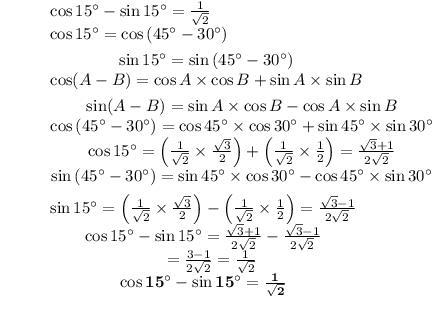QUESTION: 3

### If 2tan−1(cos x) = tan−1(2cosec x) , then x =

Solution:

If 2 tan-1 (cos x) = tan -1(2 cosec x),

2tan-1(cos x) = tan-1 (2 cosec x)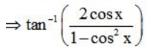= tan-1(2 cosec x)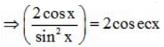= cot x cosec x = cosec x = x = π/4

QUESTION: 4

tan−1(−2) + tan−1(−3) is equal to

Solution:

tan-1(-2) + tan-1(-3)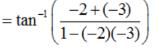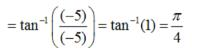QUESTION: 5

The values of x which satisfy the trigonometric equation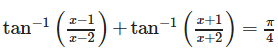are :

Solution: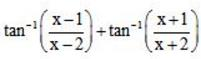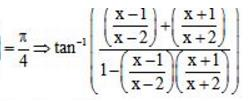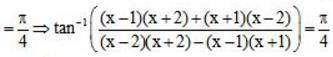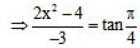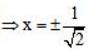QUESTION: 6

The maximum value of sin x + cos x is

Solution: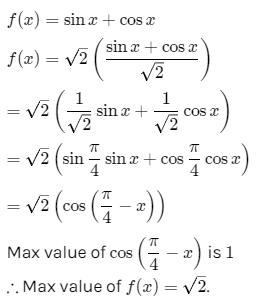QUESTION: 7

The value of tan15+ cot15is

Solution:

The value of tan150 + cot 150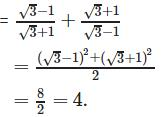QUESTION: 8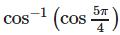is equal to

Solution: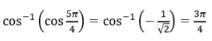QUESTION: 9

The number of solutions of the equation sin-1 x - cos-1 x = sin-1(1/2) is

Solution: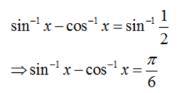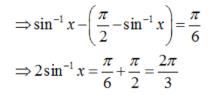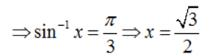Hence , the given equation has only one solution.

QUESTION: 10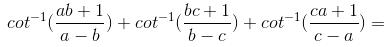Solution: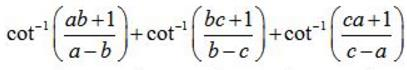cot-1a - cot-1b + cot-1 b - cot-1 c - cot-1 a = 0

QUESTION: 11

What is the maximum and minimum value of sin x +cos x?

Solution:

Let y= sin x + cos x

dy/dx=cos x- sin x

For maximum or minimum dy/dx=0

Setting cosx- sin x=0

We get cos x = sin x

x= π/4, 5π/4———-

Whether these correspond to maximum or minimum, can be found from the sign of second derivative.

d^2y/dx^2=-sin x - cos x=-1/√2–1/√2 (for x=π/4) which is negative. Hence x=π/4 corresponds to maximum.For x=5π/4

d^2y/dx^2=-(-1/√2)-(-1/√2)=2/√2 a positive quantity. Hence 5π/4 corresponds to minimum

Maximum value of the function

y= sin π/4 + cos π/4= 2/√2=√2

Minimum value is

Sin(5π/4)+cos (5π/4)=-2/√2=-√2

QUESTION: 12

sin (200)0 + cos (200)0 is

Solution:

Because both sin 2000 and cos 2000 lies in 3rd quadrant. In 3rd quadrant values of sin and cos are negative.

QUESTION: 13

If cos(-1)x + cos(-1)y = 2π, then the value of sin(-1)x + sin(-1)y is

Solution:

If cos(-1)x + cos(-1) y = 2π, then the value of sin(-1)x + sin(-1)y  = π−2π = −π.

QUESTION: 14

Domain of f(x) = sin−1x−sec−1x is

Solution:

Since sin−1x is defined for |x|⩽1, and sec−1x is defined for |x|⩾1,therefore,f(x) is defined only when|x|=1.so, Df = {−1,1}.

QUESTION: 15

The value of sin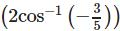is

Solution:

Put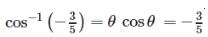therefore the given expressionis sin2θ = 2sinθcosθ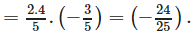QUESTION: 16

If 5 sin θ = 3, then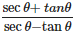is equal to

Solution: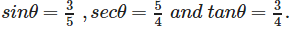= [(5/4) + (3/4)] / [(5/4) - (3/4)]
=(8/4) / (2/4)
=4

QUESTION: 17

If sin A + cos A = 1, then sin 2A is equal to

Solution:

(sinA+cosA)2
= sin2A+cos2A+2sinAcosA
=11+sin2A=1sin2A=0.
(because Sin 2A = 2sin A cos A)

QUESTION: 18

If θ = cos-1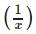, then tan θ is equal to

Solution: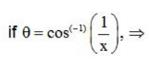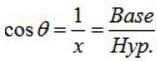Therefore, tanθ =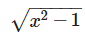QUESTION: 19

The number of solutions of the equation cos-1(1-x) - 2cos-1 x = π/2 is

Solution:

As no value of x in (0, 1) can satisfy the given equation.Thus, the given equation has only one solution.

QUESTION: 20

tan (sin−1x) is equal to

Solution: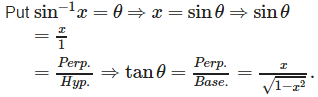QUESTION: 21

If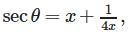x ∈ R, x ≠ 0, then the value of sec θ + tan θ is

Solution: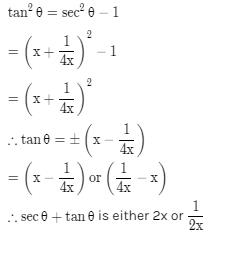QUESTION: 22

The value of cos 1050 is

Solution: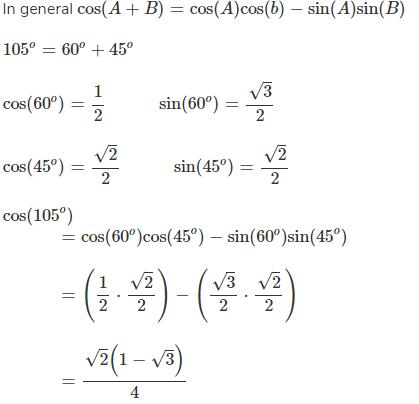QUESTION: 23

If cos(2sin−1x) = 1/9 then x =

Solution:

Put

sin-1 x = θ ⇒ x = sin θ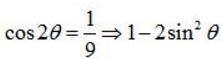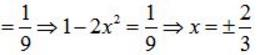QUESTION: 24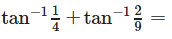Solution: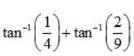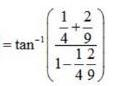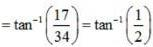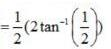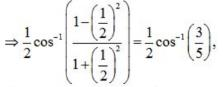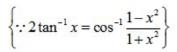QUESTION: 25

cot (cos−1x) is equal to

Solution:

Put,
cos-1x = θ ⇒ x = cos θ ⇒ cos θ